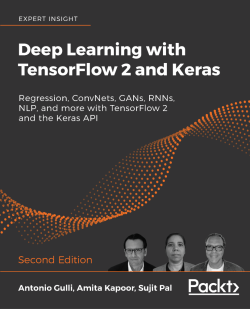# Deep Learning with TensorFlow 2 and Keras - Second Edition4.7 (6 reviews total)
By Antonio Gulli , Amita Kapoor , Sujit Pal
• \$28.99 eBook
• \$41.99 Print + eBook
What do you get with a Packt Subscription?

• Instant access to this title and 7,500+ eBooks & Videos
• Constantly updated with 100+ new titles each month
• Breadth and depth in over 1,000+ technologies

Deep Learning with TensorFlow 2 and Keras, Second Edition teaches neural networks and deep learning techniques alongside TensorFlow (TF) and Keras. You’ll learn how to write deep learning applications in the most powerful, popular, and scalable machine learning stack available.

TensorFlow is the machine learning library of choice for professional applications, while Keras offers a simple and powerful Python API for accessing TensorFlow. TensorFlow 2 provides full Keras integration, making advanced machine learning easier and more convenient than ever before.

This book also introduces neural networks with TensorFlow, runs through the main applications (regression, ConvNets (CNNs), GANs, RNNs, NLP), covers two working example apps, and then dives into TF in production, TF mobile, and using TensorFlow with AutoML.

Publication date:
December 2019
Publisher
Packt
Pages
646
ISBN
9781838823412

# Neural Network Foundations with TensorFlow 2.0

In this chapter we learn the basics of TensorFlow, an open source library developed by Google for machine learning and deep learning. In addition, we introduce the basics of neural networks and deep learning, two areas of machine learning that have had incredible Cambrian growth during the last few years. The idea behind this chapter is to give you all the tools needed to do basic but fully hands-on deep learning.

# What is TensorFlow (TF)?

TensorFlow is a powerful open source software library developed by the Google Brain team for deep neural networks, the topic covered in this book. It was first made available under the Apache 2.0 License in November 2015 and has since grown rapidly; as of May 2019, its GitHub repository (https://github.com/tensorflow/tensorflow) has more than 51,000 commits, with roughly 1,830 contributors. This in itself provides a measure of the popularity of TensorFlow.

Let us first learn what exactly TensorFlow is and why it is so popular among deep neural network researchers and engineers. Google calls it "an open source software library for machine intelligence," but since there are so many other deep learning libraries like PyTorch (https://pytorch.org/), Caffe (https://caffe.berkeleyvision.org/), and MxNet (https://mxnet.apache.org/), what makes TensorFlow special? Most other deep learning libraries – like TensorFlow – have auto-differentiation (a useful mathematical tool used for optimization), many are open source platforms, most of them support the CPU/GPU option, have pretrained models, and support commonly used NN architectures like recurrent neural networks, convolutional neural networks, and deep belief networks.

So, what else is there in TensorFlow? Let me list the top features:

• It works with all popular languages such as Python, C++, Java, R, and Go.
• Keras – a high-level neural network API that has been integrated with TensorFlow (in 2.0, Keras became the standard API for interacting with TensorFlow). This API specifies how software components should interact.
• TensorFlow allows model deployment and ease of use in production.
• Support for eager computation (see Chapter 2, TensorFlow 1.x and 2.x) has been introduced in TensorFlow 2.0, in addition to graph computation based on static graphs.
• Most importantly, TensorFlow has very good community support.

The number of stars on GitHub (see Figure 1) is a measure of popularity for all open source projects. As of March 2019, TensorFlow, Keras, and PyTorch have 123,000, 39,000, and 25,000 stars respectively, which makes TensorFlow the most popular framework for machine learning:

Figure 1: Number of stars for various deep learning projects on GitHub

Google Trends is another measure of popularity, and again TensorFlow and Keras are the two top frameworks (late 2019), with PyTorch rapidly catching up (see Figure 2).

Figure 2: Google Trends for various deep learning projects

# What is Keras?

Keras is a beautiful API for composing building blocks to create and train deep learning models. Keras can be integrated with multiple deep learning engines including Google TensorFlow, Microsoft CNTK, Amazon MxNet, and Theano. Starting with TensorFlow 2.0, Keras has been adopted as the standard high-level API, largely simplifying coding and making programming more intuitive.

# What are the most important changes in TensorFlow 2.0?

There are many changes in TensorFlow 2.0. There is no longer a need to question "Do I use Keras or TensorFlow?" because Keras is now part of TensorFlow. Another question is "Should I use Keras or `tf.keras`?" `tf.keras` is the implementation of Keras inside TensorFlow. Use `tf.keras` instead of Keras for better integration with other TensorFlow APIs, such as eager execution, `tf.data`, and many more benefits that we are going to discuss in Chapter 2, TensorFlow 1.x and 2.x.

For now, let's start with a simple code comparison just to give you some initial intuition. If you have never installed TensorFlow before, then let's install it using pip:

You can find more options for installing TensorFlow at https://www.tensorflow.org/install.

Only CPU support:

``````pip install tensorflow
``````

With GPU support:

``````pip install tensorflow-gpu
``````

In order to understand what's new in TensorFlow 2.0, it might be useful to have a look at the traditional way of coding neural networks in TensorFlow 1.0. If this is the first time you have seen a neural network, please do not pay attention to the details but simply count the number of lines:

``````import tensorflow.compat.v1 as tf
in_a = tf.placeholder(dtype=tf.float32, shape=(2))
def model(x):
with tf.variable_scope("matmul"):
W = tf.get_variable("W", initializer=tf.ones(shape=(2,2)))
b = tf.get_variable("b", initializer=tf.zeros(shape=(2)))
return x * W + b
out_a = model(in_a)
with tf.Session() as sess:
sess.run(tf.global_variables_initializer())
outs = sess.run([out_a],
feed_dict={in_a: [1, 0]})
``````

In total, we have 11 lines here. Now let's install TensorFlow 2.0:

Only CPU support:

``````pip install tensorflow==2.0.0-alpha0
``````

With GPU support:

``````pip install tensorflow-gpu==2.0.0-alpha0
``````

Here's how the code is written in TensorFlow 2.0 to achieve the same results:

``````import tensorflow as tf
W = tf.Variable(tf.ones(shape=(2,2)), name="W")
b = tf.Variable(tf.zeros(shape=(2)), name="b")
@tf.function
def model(x):
return W * x + b
out_a = model([1,0])
print(out_a)
``````

In this case, we have eight lines in total and the code looks cleaner and nicer. Indeed, the key idea of TensorFlow 2.0 is to make TensorFlow easier to learn and to apply. If you have started with TensorFlow 2.0 and have never seen TensorFlow 1.x, then you are lucky. If you are already familiar with 1.x, then it is important to understand the differences and you need to be ready to rewrite your code with some help from automatic tools for migration, as discussed in Chapter 2, TensorFlow 1.x and 2.x. Before that, let's start by introducing neural networks–one of the most powerful learning paradigms supported by TensorFlow.

# Introduction to neural networks

Artificial neural networks (briefly, "nets" or ANNs) represent a class of machine learning models loosely inspired by studies about the central nervous systems of mammals. Each ANN is made up of several interconnected "neurons," organized in "layers." Neurons in one layer pass messages to neurons in the next layer (they "fire," in jargon terms) and this is how the network computes things. Initial studies were started in the early 50's with the introduction of the "perceptron" , a two-layer network used for simple operations, and further expanded in the late 60's with the introduction of the "back-propagation" algorithm used for efficient multi-layer network training (according to , ).

Some studies argue that these techniques have roots dating further back than normally cited.

Neural networks were a topic of intensive academic studies up until the 80's, at which point other, simpler approaches became more relevant. However, there has been a resurgence of interest starting in the mid 2000's, mainly thanks to three factors: a breakthrough fast learning algorithm proposed by G. Hinton , , ; the introduction of GPUs around 2011 for massive numeric computation; and the availability of big collections of data for training.

These improvements opened the route for modern "deep learning," a class of neural networks characterized by a significant number of layers of neurons that are able to learn rather sophisticated models based on progressive levels of abstraction. People began referring to it as "deep" when it started utilizing 3-5 layers a few years ago. Now, networks with more than 200 layers are commonplace!

This learning via progressive abstraction resembles vision models that have evolved over millions of years within the human brain. Indeed, the human visual system is organized into different layers. First, our eyes are connected to an area of the brain named the visual cortex (V1), which is located in the lower posterior part of our brain. This area is common to many mammals and has the role of discriminating basic properties like small changes in visual orientation, spatial frequencies, and colors.

It has been estimated that V1 consists of about 140 million neurons, with tens of billions of connections between them. V1 is then connected to other areas (V2, V3, V4, V5, and V6) doing progressively more complex image processing and recognizing more sophisticated concepts, such as shapes, faces, animals, and many more. It has been estimated that there are ~16 billion human cortical neurons and about 10-25% of the human cortex is devoted to vision . Deep learning has taken some inspiration from this layer-based organization of the human visual system: early artificial neuron layers learn basic properties of images while deeper layers learn more sophisticated concepts.

This book covers several major aspects of neural networks by providing working nets in TensorFlow 2.0. So, let's start!

# Perceptron

The "perceptron" is a simple algorithm that, given an input vector x of m values (x1, x2,..., xm), often called input features or simply features, outputs either a 1 ("yes") or a 0 ("no"). Mathematically, we define a function:

Where w is a vector of weights, wx is the dot product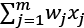and b is bias. If you remember elementary geometry, wx + b defines a boundary hyperplane that changes position according to the values assigned to w and b.

Note that a hyperplane is a subspace whose dimension is one less than that of its ambient space. See Figure 3 for an example:

Figure 3: An example of a hyperplane

In other words, this is a very simple but effective algorithm! For example, given three input features, the amounts of red, green, and blue in a color, the perceptron could try to decide whether the color is white or not.

Note that the perceptron cannot express a "maybe" answer. It can answer "yes" (1) or "no" (0), if we understand how to define w and b. This is the "training" process that will be discussed in the following sections.

## A first example of TensorFlow 2.0 code

There are three ways of creating a model in `tf.keras`: Sequential API , Functional API, and Model subclassing. In this chapter we will use the simplest one, `Sequential()`, while the other two are discussed in Chapter 2, TensorFlow 1.x and 2.x. A `Sequential()` model is a linear pipeline (a stack) of neural network layers. This code fragment defines a single layer with 10 artificial neurons that expects 784 input variables (also known as features). Note that the net is "dense," meaning that each neuron in a layer is connected to all neurons located in the previous layer, and to all the neurons in the following layer:

``````import tensorflow as tf
from tensorflow import keras
NB_CLASSES = 10
RESHAPED = 784
model = tf.keras.models.Sequential()
input_shape=(RESHAPED,), kernel_initializer='zeros',
name='dense_layer', activation='softmax'))
``````

Each neuron can be initialized with specific weights via the `kernel_initializer` parameter. There are a few choices, the most common of which are listed as follows:

• `random_uniform`: Weights are initialized to uniformly random small values in the range -0.05 to 0.05.
• `random_normal`: Weights are initialized according to a Gaussian distribution, with zero mean and a small standard deviation of 0.05. For those of you who are not familiar with Gaussian distribution, think about a symmetric "bell curve" shape.
• `zero`: All weights are initialized to zero.

A full list is available online at https://www.tensorflow.org/api_docs/python/tf/keras/initializers.

# Multi-layer perceptron – our first example of a network

In this chapter, we present our first example of a network with multiple dense layers. Historically, "perceptron" was the name given to a model having one single linear layer, and as a consequence, if it has multiple layers, you would call it a multi-layer perceptron (MLP). Note that the input and the output layers are visible from outside, while all the other layers in the middle are hidden – hence the name hidden layers. In this context, a single layer is simply a linear function and the MLP is therefore obtained by stacking multiple single layers one after the other:

Figure 4: An example of a multiple layer perceptron

In Figure 4 each node in the first hidden layer receives an input and "fires" (0,1) according to the values of the associated linear function. Then, the output of the first hidden layer is passed to the second layer where another linear function is applied, the results of which are passed to the final output layer consisting of one single neuron. It is interesting to note that this layered organization vaguely resembles the organization of the human vision system, as we discussed earlier.

## Problems in training the perceptron and their solutions

Let's consider a single neuron; what are the best choices for the weight w and the bias b? Ideally, we would like to provide a set of training examples and let the computer adjust the weight and the bias in such a way that the errors produced in the output are minimized.

In order to make this a bit more concrete, let's suppose that we have a set of images of cats and another separate set of images not containing cats. Suppose that each neuron receives input from the value of a single pixel in the images. While the computer processes those images, we would like our neuron to adjust its weights and its bias so that we have fewer and fewer images wrongly recognized.

This approach seems very intuitive, but it requires a small change in the weights (or the bias) to cause only a small change in the outputs. Think about it: if we have a big output jump, we cannot learn progressively. After all, kids learn little by little. Unfortunately, the perceptron does not show this "little-by-little" behavior. A perceptron is either a 0 or 1, and that's a big jump that will not help in learning (see Figure 5):

Figure 5: Example of perceptron - either a 0 or 1

We need something different; something smoother. We need a function that progressively changes from 0 to 1 with no discontinuity. Mathematically, this means that we need a continuous function that allows us to compute the derivative. You might remember that in mathematics the derivative is the amount by which a function changes at a given point. For functions with input given by real numbers, the derivative is the slope of the tangent line at a point on a graph. Later in this chapter, we will see why derivatives are important for learning, when we talk about gradient descent.

## Activation function – sigmoid

The sigmoid function defined as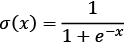and represented in the following figure has small output changes in the range (0, 1) when the input varies in the range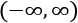. Mathematically the function is continuous. A typical sigmoid function is represented in Figure 6:

Figure 6: A sigmoid function with output in the range (0,1)

A neuron can use the sigmoid for computing the nonlinear function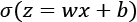. Note that if z = wx + b is very large and positive, then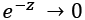so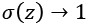, while if z = wx + b is very large and negative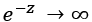so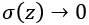. In other words, a neuron with sigmoid activation has a behavior similar to the perceptron, but the changes are gradual and output values such as 0.5539 or 0.123191 are perfectly legitimate. In this sense, a sigmoid neuron can answer "maybe."

## Activation function – tanh

Another useful activation function is tanh. Defined aswhose shape is shown in Figure 7, its outputs range from -1 to 1:

Figure 7: Tanh activation function

## Activation function – ReLU

The sigmoid is not the only kind of smooth activation function used for neural networks. Recently, a very simple function named ReLU (REctified Linear Unit) became very popular because it helps address some optimization problems observed with sigmoids. We will discuss these problems in more detail when we talk about vanishing gradient in Chapter 9, Autoencoders. A ReLU is simply defined as f(x) = max(0, x) and the non-linear function is represented in Figure 8. As you can see, the function is zero for negative values and it grows linearly for positive values. The ReLU is also very simple to implement (generally, three instructions are enough), while the sigmoid is a few orders of magnitude more. This helped to squeeze the neural networks onto an early GPU:

Figure 8: A ReLU function

## Two additional activation functions – ELU and LeakyReLU

Sigmoid and ReLU are not the only activation functions used for learning.

ELU is defined as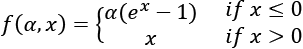for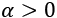and its plot is represented in Figure 9:

Figure 9: An ELU function

LeakyReLU is defined as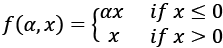for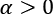and its plot is represented in Figure 10:

Figure 10: A LeakyReLU function

Both the functions allow small updates if x is negative, which might be useful in certain conditions.

## Activation functions

Sigmoid, Tanh, ELU, LeakyReLU, and ReLU are generally called activation functions in neural network jargon. In the gradient descent section, we will see that those gradual changes typical of sigmoid and ReLU functions are the basic building blocks to develop a learning algorithm that adapts little by little by progressively reducing the mistakes made by our nets. An example of using the activation function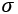with (x1, x2,..., xm) input vector, (w1, w2,..., wm) weight vector, b bias, and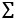summation is given in Figure 11. Note that TensorFlow 2.0 supports many activation functions, a full list of which is available online:

Figure 11: An example of an activation function applied after a linear function

## In short – what are neural networks after all?

In one sentence, machine learning models are a way to compute a function that maps some inputs to their corresponding outputs. The function is nothing more than a number of addition and multiplication operations. However, when combined with a non-linear activation and stacked in multiple layers, these functions can learn almost anything . You also need a meaningful metric capturing what you want to optimize (this being the so-called loss function that we will cover later in the book), enough data to learn from, and sufficient computational power.

Now, it might be beneficial to stop one moment and ask ourselves what "learning" really is? Well, we can say for our purposes that learning is essentially a process aimed at generalizing established observations  in order to predict future results. So, in short, this is exactly the goal we want to achieve with neural networks.

# A real example – recognizing handwritten digits

In this section we will build a network that can recognize handwritten numbers. In order to achieve this goal, we'll use MNIST (http://yann.lecun.com/exdb/mnist/), a database of handwritten digits made up of a training set of 60,000 examples, and a test set of 10,000 examples. The training examples are annotated by humans with the correct answer. For instance, if the handwritten digit is the number "3", then 3 is simply the label associated with that example.

In machine learning, when a dataset with correct answers is available, we say that we can perform a form of supervised learning. In this case we can use training examples to improve our net. Testing examples also have the correct answer associated to each digit. In this case, however, the idea is to pretend that the label is unknown, let the network do the prediction, and then later on reconsider the label to evaluate how well our neural network has learned to recognize digits. Unsurprisingly, testing examples are just used to test the performance of our net.

Each MNIST image is in grayscale and consists of 28*28 pixels. A subset of these images of numbers is shown in Figure 12:

Figure 12: A collection of MNIST images

## One-hot encoding (OHE)

We are going to use OHE as a simple tool to encode information used inside neural networks. In many applications it is convenient to transform categorical (non-numerical) features into numerical variables. For instance, the categorical feature "digit" with value d in [0 – 9] can be encoded into a binary vector with 10 positions, which always has 0 value except the d - th position where a 1 is present.

For example, the digit 3 can be encoded as [0, 0, 0, 1, 0, 0, 0, 0, 0, 0]. This type of representation is called One-hot encoding, or sometimes simply one-hot, and is very common in data mining when the learning algorithm is specialized in dealing with numerical functions.

## Defining a simple neural network in TensorFlow 2.0

In this section, we use TensorFlow 2.0 to define a network that recognizes MNIST handwritten digits. We start with a very simple neural network and then progressively improve it.

Following Keras style, TensorFlow 2.0 provides suitable libraries (https://www.tensorflow.org/api_docs/python/tf/keras/datasets) for loading the dataset and splits it into training sets, `X_train`, used for fine-tuning our net, and test sets, `X_test`, used for assessing the performance. Data is converted into `float32` to use 32-bit precision when training a neural network and normalized to the range [0,1]. In addition, we load the true labels into `Y_train` and `Y_test` respectively, and perform a one-hot encoding on them. Let's see the code.

For now, do not focus too much on understanding why certain parameters have specific assigned values, as these choices will be discussed throughout the rest of the book. Intuitively, `EPOCH` defines how long the training should last, `BATCH_SIZE` is the number of samples you feed in to your network at a time, and `VALIDATION` is the amount of data reserved for checking or proving the validity of the training process. The reason why we picked `EPOCHS = 200`, `BATCH_SIZE = 128`, `VALIDATION_SPLIT=0.2`, and `N_HIDDEN = 128` will be clearer later in this chapter when we will explore different values and discuss hyperparameter optimization. Let's look at our first code fragment of a neural network in TensorFlow. Reading is intuitive but you will find a detailed explanation in the following pages:

``````import tensorflow as tf
import numpy as np
from tensorflow import keras
# Network and training parameters.
EPOCHS = 200
BATCH_SIZE = 128
VERBOSE = 1
NB_CLASSES = 10   # number of outputs = number of digits
N_HIDDEN = 128
VALIDATION_SPLIT = 0.2 # how much TRAIN is reserved for VALIDATION
# verify
# You can verify that the split between train and test is 60,000, and 10,000 respectively.
# Labels have one-hot representation.is automatically applied
mnist = keras.datasets.mnist
(X_train, Y_train), (X_test, Y_test) = mnist.load_data()
# X_train is 60000 rows of 28x28 values; we  --> reshape it to
# 60000 x 784.
RESHAPED = 784
#
X_train = X_train.reshape(60000, RESHAPED)
X_test = X_test.reshape(10000, RESHAPED)
X_train = X_train.astype('float32')
X_test = X_test.astype('float32')
# Normalize inputs to be within in [0, 1].
X_train /= 255
X_test /= 255
print(X_train.shape, 'train samples')
print(X_test.shape, 'test samples')
# One-hot representation of the labels.
Y_train = tf.keras.utils.to_categorical(Y_train, NB_CLASSES)
Y_test = tf.keras.utils.to_categorical(Y_test, NB_CLASSES)
``````

You can see from the above code that the input layer has a neuron associated to each pixel in the image for a total of 28*28=784 neurons, one for each pixel in the MNIST images.

Typically, the values associated with each pixel are normalized in the range [0,1] (which means that the intensity of each pixel is divided by 255, the maximum intensity value). The output can be one of ten classes, with one class for each digit.

The final layer is a single neuron with activation function "softmax", which is a generalization of the sigmoid function. As discussed earlier, a sigmoid function output is in the range (0, 1) when the input varies in the range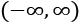. Similarly, a softmax "squashes" a K-dimensional vector of arbitrary real values into a K-dimensional vector of real values in the range (0, 1), so that they all add up to 1. In our case, it aggregates 10 answers provided by the previous layer with 10 neurons. What we have just described is implemented with the following code:

``````# Build the model.
model = tf.keras.models.Sequential()
input_shape=(RESHAPED,),
name='dense_layer',
activation='softmax'))
``````

Once we define the model, we have to compile it so that it can be executed by TensorFlow 2.0. There are a few choices to be made during compilation. Firstly, we need to select an optimizer, which is the specific algorithm used to update weights while we train our model. Second, we need to select an objective function, which is used by the optimizer to navigate the space of weights (frequently, objective functions are called either loss functions or cost functions and the process of optimization is defined as a process of loss minimization). Third, we need to evaluate the trained model.

A complete list of optimizers can be found at https://www.tensorflow.org/api_docs/python/tf/keras/optimizers.

Some common choices for objective functions are:

• `MSE`, which defines the mean squared error between the predictions and the true values. Mathematically, if d is a vector of predictions and y is the vector of n observed values, then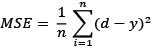. Note that this objective function is the average of all the mistakes made in each prediction. If a prediction is far off from the true value, then this distance is made more evident by the squaring operation. In addition, the square can add up the error regardless of whether a given value is positive or negative.
• `binary_crossentropy`, which defines the binary logarithmic loss. Suppose that our model predicts p while the target is c, then the binary cross-entropy is defined as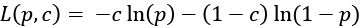. Note that this objective function is suitable for binary label prediction.
• `categorical_crossentropy`, which defines the multiclass logarithmic loss. Categorical cross-entropy compares the distribution of the predictions with the true distribution, with the probability of the true class set to 1 and 0 for the other classes. If the true class is c and the prediction is y, then the categorical cross-entropy is defined as:

One way to think about multi-class logarithm loss is to consider the true class represented as a one-hot encoded vector, and the closer the model's outputs are to that vector, the lower the loss. Note that this objective function is suitable for multi-class label predictions. It is also the default choice in association with softmax activation.

A complete list of loss functions can be found at https://www.tensorflow.org/api_docs/python/tf/keras/losses.

Some common choices for metrics are:

• `Accuracy`, which defines the proportion of correct predictions with respect to the targets
• `Precision`, which defines how many selected items are relevant for a multi-label classification
• `Recall`, which defines how many selected items are relevant for a multi-label classification

A complete list of metrics can be found at https://www.tensorflow.org/api_docs/python/tf/keras/metrics.

Metrics are similar to objective functions, with the only difference that they are not used for training a model, but only for evaluating the model. However, it is important to understand the difference between metrics and objective functions. As discussed, the loss function is used to optimize your network. This is the function minimized by the selected optimizer. Instead, a metric is used to judge the performance of your network. This is only for you to run an evaluation on and it should be separated from the optimization process. On some occasions, it would be ideal to directly optimize for a specific metric. However, some metrics are not differentiable with respect to their inputs, which precludes them from being used directly.

When compiling a model in TensorFlow 2.0, it is possible to select the optimizer, the loss function, and the metric used together with a given model:

``````# Compiling the model.
model.compile(optimizer='SGD',
loss='categorical_crossentropy',
metrics=['accuracy'])
``````

Stochastic Gradient Descent (SGD) (see Chapter 15, The Math Behind Deep Learning) is a particular kind of optimization algorithm used to reduce the mistakes made by neural networks after each training epoch. We will review SGD and other optimization algorithms in the next chapters. Once the model is compiled, it can then be trained with the `fit()` method, which specifies a few parameters:

• `epochs` is the number of times the model is exposed to the training set. At each iteration the optimizer tries to adjust the weights so that the objective function is minimized.
• `batch_size` is the number of training instances observed before the optimizer performs a weight update; there are usually many batches per epoch.

Training a model in TensorFlow 2.0 is very simple:

``````# Training the model.
model.fit(X_train, Y_train,
batch_size=BATCH_SIZE, epochs=EPOCHS,
verbose=VERBOSE, validation_split=VALIDATION_SPLIT)
``````

Note that we've reserved part of the training set for validation. The key idea is that we reserve a part of the training data for measuring the performance on the validation while training. This is a good practice to follow for any machine learning task, and one that we will adopt in all of our examples. Please note that we will return to validation later in this chapter when we talk about overfitting.

Once the model is trained, we can evaluate it on the test set that contains new examples never seen by the model during the training phase.

Note that, of course, the training set and the test set are rigorously separated. There is no point evaluating a model on an example that was already used for training. In TensorFlow 2.0 we can use the method `evaluate(X_test, Y_test)` to compute the `test_loss` and the `test_acc`:

``````#evaluate the model
test_loss, test_acc = model.evaluate(X_test, Y_test)
print('Test accuracy:', test_acc)
``````

So, congratulations! You have just defined your first neural network in TensorFlow 2.0. A few lines of code and your computer should be able to recognize handwritten numbers. Let's run the code and see what the performance is.

## Running a simple TensorFlow 2.0 net and establishing a baseline

So let's see what happens when we run the code:

Figure 13: Code ran from our test neural network

First, the net architecture is dumped and we can see the different types of layers used, their output shape, how many parameters (that is, how many weights) they need to optimize, and how they are connected. Then, the network is trained on 48,000 samples, and 12,000 are reserved for validation. Once the neural model is built, it is then tested on 10,000 samples. For now, we won't go into the internals of how the training happens, but we can see that the program runs for 200 iterations and each time accuracy improves. When the training ends, we test our model on the test set and we achieve about 89.96% accuracy on training, 90.70% on validation, and 90.71% on test:

Figure 14: Results from testing model, accuracies displayed

This means that nearly 1 in 10 images are incorrectly classified. We can certainly do better than that.

## Improving the simple net in TensorFlow 2.0 with hidden layers

Okay, we have a baseline of accuracy of 89.96% on training, 90.70% on validation, and 90.71% on test. It is a good starting point, but we can improve it. Let's see how.

An initial improvement is to add additional layers to our network because these additional neurons might intuitively help it to learn more complex patterns in the training data. In other words, additional layers add more parameters, potentially allowing a model to memorize more complex patterns. So, after the input layer, we have a first dense layer with `N_HIDDEN` neurons and an activation function "ReLU." This additional layer is considered hidden because it is not directly connected either with the input or with the output. After the first hidden layer, we have a second hidden layer again with `N_HIDDEN` neurons followed by an output layer with 10 neurons, each one of which will fire when the relative digit is recognized. The following code defines this new network:

``````import tensorflow as tf
from tensorflow import keras
# Network and training.
EPOCHS = 50
BATCH_SIZE = 128
VERBOSE = 1
NB_CLASSES = 10   # number of outputs = number of digits
N_HIDDEN = 128
VALIDATION_SPLIT = 0.2 # how much TRAIN is reserved for VALIDATION
# Labels have one-hot representation.
mnist = keras.datasets.mnist
(X_train, Y_train), (X_test, Y_test) = mnist.load_data()
# X_train is 60000 rows of 28x28 values; we reshape it to 60000 x 784.
RESHAPED = 784
#
X_train = X_train.reshape(60000, RESHAPED)
X_test = X_test.reshape(10000, RESHAPED)
X_train = X_train.astype('float32')
X_test = X_test.astype('float32')
# Normalize inputs to be within in [0, 1].
X_train, X_test = X_train / 255.0, X_test / 255.0
print(X_train.shape, 'train samples')
print(X_test.shape, 'test samples')
# Labels have one-hot representation.
Y_train = tf.keras.utils.to_categorical(Y_train, NB_CLASSES)
Y_test = tf.keras.utils.to_categorical(Y_test, NB_CLASSES)
# Build the model.
model = tf.keras.models.Sequential()
input_shape=(RESHAPED,),
name='dense_layer', activation='relu'))
name='dense_layer_2', activation='relu'))
name='dense_layer_3', activation='softmax'))
# Summary of the model.
model.summary()
# Compiling the model.
model.compile(optimizer='SGD',
loss='categorical_crossentropy',
metrics=['accuracy'])
# Training the model.
model.fit(X_train, Y_train,
batch_size=BATCH_SIZE, epochs=EPOCHS,
verbose=VERBOSE, validation_split=VALIDATION_SPLIT)
# Evaluating the model.
test_loss, test_acc = model.evaluate(X_test, Y_test)
print('Test accuracy:', test_acc)
``````

Note that `to_categorical(Y_train, NB_CLASSES)` converts the array `Y_train` into a matrix with as many columns as there are classes. The number of rows stays the same. So, for instance if we have:

``````> labels
array([0, 2, 1, 2, 0])
``````

then:

``````to_categorical(labels)
array([[ 1.,  0.,  0.],
[ 0.,  0.,  1.],
[ 0.,  1.,  0.],
[ 0.,  0.,  1.],
[ 1.,  0.,  0.]], dtype=float32)
``````

Let's run the code and see what results we get with this multi-layer network:

Figure 15: Running the code for a multi-layer network

The previous screenshot shows the initial steps of the run while the following screenshot shows the conclusion. Not bad. As seen in the following screenshot, by adding two hidden layers we reached 90.81% on the training set, 91.40% on validation, and 91.18% on test. This means that we have increased accuracy on testing with respect to the previous network, and we have reduced the number of iterations from 200 to 50. That's good, but we want more.

If you want, you can play by yourself and see what happens if you add only one hidden layer instead of two or if you add more than two layers. I leave this experiment as an exercise:

Figure 16: Results after adding two hidden layers, with accuracies shown

Note that improvement stops (or they become almost imperceptible) after a certain number of epochs. In machine learning, this is a phenomenon called convergence.

## Further improving the simple net in TensorFlow with Dropout

Now our baseline is 90.81% on the training set, 91.40% on validation, and 91.18% on test. A second improvement is very simple. We decide to randomly drop – with the `DROPOUT` probability – some of the values propagated inside our internal dense network of hidden layers during training. In machine learning this is a well-known form of regularization. Surprisingly enough, this idea of randomly dropping a few values can improve our performance. The idea behind this improvement is that random dropout forces the network to learn redundant patterns that are useful for better generalization:

``````import tensorflow as tf
import numpy as np
from tensorflow import keras
# Network and training.
EPOCHS = 200
BATCH_SIZE = 128
VERBOSE = 1
NB_CLASSES = 10   # number of outputs = number of digits
N_HIDDEN = 128
VALIDATION_SPLIT = 0.2 # how much TRAIN is reserved for VALIDATION
DROPOUT = 0.3
# Labels have one-hot representation.
mnist = keras.datasets.mnist
(X_train, Y_train), (X_test, Y_test) = mnist.load_data()
# X_train is 60000 rows of 28x28 values; we reshape it to 60000 x 784.
RESHAPED = 784
#
X_train = X_train.reshape(60000, RESHAPED)
X_test = X_test.reshape(10000, RESHAPED)
X_train = X_train.astype('float32')
X_test = X_test.astype('float32')
# Normalize inputs within [0, 1].
X_train, X_test = X_train / 255.0, X_test / 255.0
print(X_train.shape, 'train samples')
print(X_test.shape, 'test samples')
# One-hot representations for labels.
Y_train = tf.keras.utils.to_categorical(Y_train, NB_CLASSES)
Y_test = tf.keras.utils.to_categorical(Y_test, NB_CLASSES)
# Building the model.
model = tf.keras.models.Sequential()
input_shape=(RESHAPED,),
name='dense_layer', activation='relu'))
name='dense_layer_2', activation='relu'))
name='dense_layer_3', activation='softmax'))
# Summary of the model.
model.summary()
# Compiling the model.
model.compile(optimizer='SGD',
loss='categorical_crossentropy',
metrics=['accuracy'])
# Training the model.
model.fit(X_train, Y_train,
batch_size=BATCH_SIZE, epochs=EPOCHS,
verbose=VERBOSE, validation_split=VALIDATION_SPLIT)
# Evaluating the model.
test_loss, test_acc = model.evaluate(X_test, Y_test)
print('Test accuracy:', test_acc)
``````

Let's run the code for 200 iterations as before, and we'll see that this net achieves an accuracy of 91.70% on training, 94.42% on validation, and 94.15% on testing:

Figure 17: Further testing of the neutal network, with accuracies shown

Note that it has been frequently observed that networks with random dropout in internal hidden layers can "generalize" better on unseen examples contained in test sets. Intuitively, we can consider this phenomenon as each neuron becoming more capable because it knows it cannot depend on its neighbors. Also, because it forces information to be stored in a redundant way. During testing there is no dropout, so we are now using all our highly tuned neurons. In short, it is generally a good approach to test how a net performs when a dropout function is adopted.

Besides that, note that training accuracy should still be above test accuracy, otherwise, we might be not training for long enough. This is the case in our example and therefore we should increase the number of epochs. However, before performing this attempt we need to introduce a few other concepts that allow the training to converge faster. Let's talk about optimizers.

## Testing different optimizers in TensorFlow 2.0

Now that we have defined and used a network, it is useful to start developing some intuition about how networks are trained, using an analogy. Let us focus on one popular training technique known as Gradient Descent (GD). Imagine a generic cost function C(w) in one single variable w as shown in Figure 18:

Figure 18: An example of gradient descent optimization

The gradient descent can be seen as a hiker who needs to navigate down a steep slope and aims to enter a ditch. The slope represents the function C while the ditch represents the minimum Cmin. The hiker has a starting point w0. The hiker moves little by little; imagine that there is almost zero visibility, so the hiker cannot see where to go automatically, and they proceed in a zigzag. At each step r, the gradient is the direction of maximum increase.

Mathematically this direction is the value of the partial derivative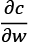evaluated at point wr, reached at step r. Therefore, by taking the opposite direction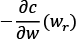the hiker can move towards the ditch.

At each step, the hiker can decide how big a stride to take before the next stop. This is the so-called "learning rate"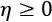in gradient descent jargon. Note that if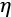is too small, then the hiker will move slowly. However, ifis too high, then the hiker will possibly miss the ditch by stepping over it.

Now you should remember that a sigmoid is a continuous function and it is possible to compute the derivative. It can be proven that the sigmoid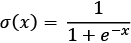has the derivative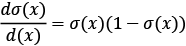.

ReLU is not differentiable at 0. We can however extend the first derivative at 0 to a function over the whole domain by defining it to be either a 0 or 1.

The piecewise derivative of ReLU y = max(0, x) is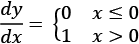. Once we have the derivative, it is possible to optimize the nets with a gradient descent technique. TensorFlow computes the derivative on our behalf so we don't need to worry about implementing or computing it.

A neural network is essentially a composition of multiple derivable functions with thousands and sometimes millions of parameters. Each network layer computes a function, the error of which should be minimized in order to improve the accuracy observed during the learning phase. When we discuss backpropagation, we will discover that the minimization game is a bit more complex than our toy example. However, it is still based on the same intuition of descending a slope to reach a ditch.

TensorFlow implements a fast variant of gradient descent known as SGD and many more advanced optimization techniques such as RMSProp and Adam. RMSProp and Adam include the concept of momentum (a velocity component), in addition to the acceleration component that SGD has. This allows faster convergence at the cost of more computation. Think about a hiker who starts to move in one direction then decides to change direction but remembers previous choices. It can be proven that momentum helps accelerate SGD in the relevant direction and dampens oscillations .

A complete list of optimizers can be found at https://www.tensorflow.org/api_docs/python/tf/keras/optimizers.

SGD was our default choice so far. So now let's try the other two.

It is very simple; we just need to change a few lines:

``````# Compiling the model.
model.compile(optimizer='RMSProp',
loss='categorical_crossentropy', metrics=['accuracy'])
``````

That's it. Let's test it:

Figure 19: Testing RMSProp

As you can see in the preceding screenshot, RMSProp is faster than SDG since we are able to achieve in only 10 epochs an accuracy of 97.43% on training, 97.62% on validation, and 97.64% on test. That's a significant improvement on SDG. Now that we have a very fast optimizer, let us try to significantly increase the number of epochs up to 250 and we get 98.99% accuracy on training, 97.66% on validation, and 97.77% on test:

Figure 20: Increasing the number of epochs

It is useful to observe how accuracy increases on training and test sets when the number of epochs increases (see Figure 21). As you can see, these two curves touch at about 15 epochs and therefore there is no need to train further after that point (the image is generated by using TensorBoard, a standard TensorFlow tool that will be discussed in Chapter 2, TensorFlow 1.x and 2.x):

Figure 21: An example of accuracy and loss with RMSProp

Okay, let's try the other optimizer, `Adam()`. Pretty simple:

``````# Compiling the model.
loss='categorical_crossentropy',
metrics=['accuracy'])
``````

As we can see, `Adam()` is slightly better. With Adam we achieve 98.94% accuracy on training, 97.89% on validation, and 97.82% on test with 20 iterations:

Figure 22: Testing with the Adam optimizer

One more time, let's plot how accuracy increases on training and test sets when the number of epochs increases (see Figure 23). You'll notice that by choosing Adam as an optimizer, we are able to stop after just about 12 epochs or steps:

Figure 23: An example of accuracy and loss with adam

Note that this is our fifth variant and remember that our initial baseline was at 90.71% on test. So far, we've made progressive improvements. However, gains are now more and more difficult to obtain. Note that we are optimizing with a dropout of 30%. For the sake of completeness, it could be useful to report the accuracy on the test dataset for different dropout values (see Figure 24). In this example, we selected `Adam()` as the optimizer. Note that choice of optimizer isn't a rule of thumb and we can get different performance depending on the problem-optimizer combination:

Figure 24: An example of changes in accuracy for different Dropout values

## Increasing the number of epochs

Let's make another attempt and increase the number of epochs used for training from 20 to 200. Unfortunately, this choice increases our computation time tenfold, yet gives us no gain. The experiment is unsuccessful, but we have learned that if we spend more time learning, we will not necessarily improve the result. Learning is more about adopting smart techniques and not necessarily about the time spent in computations. Let's keep track of our five variants in the following graph (see Figure 25):

Figure 25: Accuracy for different models and optimizers

## Controlling the optimizer learning rate

There is another approach we can take that involves changing the learning parameter for our optimizer. As you can see in Figure 26, the best value reached by our three experiments [lr=0.1, lr=0.01, lr=0.001] is 0.1, which is the default learning rate for the optimizer. Good! adam works well out of the box:

Figure 26: Accuracy for different learning rates

## Increasing the number of internal hidden neurons

Yet another approach involves changing the number of internal hidden neurons. We report the results of the experiments with an increasing number of hidden neurons. We see that by increasing the complexity of the model, the runtime increases significantly because there are more and more parameters to optimize. However, the gains that we are getting by increasing the size of the network decrease more and more as the network grows (see Figures 27, 28, and 29). Note that increasing the number of hidden neurons after a certain value can reduce the accuracy because the network might not be able to generalize well (as shown in Figure 29):

Figure 27: Number of parameters for increasing values of internal hidden neurons

Figure 28: Seconds of computation time for increasing values of internal hidden neurons

Figure 29: Test accuracy for increasing the values of internal hidden neurons

## Increasing the size of batch computation

Gradient descent tries to minimize the cost function on all the examples provided in the training sets and, at the same time, for all the features provided in input. SGD is a much less expensive variant that considers only `BATCH_SIZE` examples. So, let us see how it behaves when we change this parameter. As you can see, the best accuracy value is reached for a `BATCH_SIZE=64` in our four experiments (see Figure 30):

Figure 30: Test accuracy for different batch values

## Summarizing experiments run for recognizing handwritten charts

So, let's summarize: with five different variants, we were able to improve our performance from 90.71% to 97.82%. First, we defined a simple layer network in TensorFlow 2.0. Then, we improved the performance by adding some hidden layers. After that, we improved the performance on the test set by adding a few random dropouts in our network, and then by experimenting with different types of optimizers:

 model/accuracy training validation test simple 89.96% 90.70% 90.71% 2 hidden(128) 90.81% 91.40% 91.18% dropout(30%) 91.70% 94.42% 94.15% (200 epochs) RMSProp 97.43% 97.62% 97.64% (10 epochs) Adam 98.94% 97.89% 97.82% (10 epochs)

However, the next two experiments (not shown in the preceding table) were not providing significant improvements. Increasing the number of internal neurons creates more complex models and requires more expensive computations, but it provides only marginal gains. We have the same experience if we increase the number of training epochs. A final experiment consisted of changing the `BATCH_SIZE` for our optimizer. This also provided marginal results.

# Regularization

In this section, we will review a few best practices for improving the training phase. In particular, regularization and batch normalization will be discussed.

## Adopting regularization to avoid overfitting

Intuitively, a good machine learning model should achieve a low error rate on training data. Mathematically this is equivalent to minimizing the loss function on the training data given the model:

min: {loss(Training Data | Model)}

However, this might not be enough. A model can become excessively complex in order to capture all the relations inherently expressed by the training data. This increase of complexity might have two negative consequences. First, a complex model might require a significant amount of time to be executed. Second, a complex model might achieve very good performance on training data, but perform quite badly on validation data. This is because the model is able to contrive relationships between many parameters in the specific training context, but these relationships in fact do not exist within a more generalized context. Causing a model to lose its ability to generalize in this manner is termed "overfitting." Again, learning is more about generalization than memorization:

Figure 31: Loss function and overfitting

As a rule of thumb, if during the training we see that the loss increases on validation, after an initial decrease, then we have a problem of model complexity, which overfits to the training data.

In order to solve the overfitting problem, we need a way to capture the complexity of a model, that is, how complex a model can be. What could the solution be? Well, a model is nothing more than a vector of weights. Each weight affects the output, except for those which are zero, or very close to it. Therefore, the complexity of a model can be conveniently represented as the number of non-zero weights. In other words, if we have two models M1 and M2 achieving pretty much the same performance in terms of loss function, then we should choose the simplest model, the one which has the minimum number of non-zero weights.

We can use a hyperparameter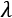>=0 for controlling the importance of having a simple model, as in this formula:

min: {loss(Training Data|Model)} +* complexity(Model)

There are three different types of regularization used in machine learning:

• L1 regularization (also known as LASSO): The complexity of the model is expressed as the sum of the absolute values of the weights.
• L2 regularization (also known as Ridge): The complexity of the model is expressed as the sum of the squares of the weights
• Elastic regularization: The complexity of the model is captured by a combination of the preceding two techniques

Note that playing with regularization can be a good way to increase the performance of a network, particularly when there is an evident situation of overfitting. This set of experiments is left as an exercise for the interested reader.

Also note that TensorFlow supports L1, L2, and ElasticNet regularization. Adding regularization is easy:

``````from tf.keras.regularizers import l2, activity_l2
activity_regularizer=activity_l2(0.01)))
``````

A complete list of regularizers can be found at https://www.tensorflow.org/api_docs/python/tf/keras/regularizers.

## Understanding BatchNormalization

BatchNormalization is another form of regularization and one of the most effective improvements proposed during the last few years. BatchNormalization enables us to accelerate training, in some cases by halving the training epochs, and it offers some regularization. Let's see what the intuition is behind it.

During training, weights in early layers naturally change and therefore the inputs of later layers can significantly change. In other words, each layer must continuously re-adjust its weights to the different distribution for every batch. This may slow down the model's training greatly. The key idea is to make layer inputs more similar in distribution, batch after batch and epoch after epoch.

Another issue is that the sigmoid activation function works very well close to zero, but tends to "get stuck" when values get sufficiently far away from zero. If, occasionally, neuron outputs fluctuate far away from the sigmoid zero, then said neuron becomes unable to update its own weights.

The other key idea is therefore to transform the layer outputs into a Gaussian distribution unit close to zero. In this way, layers will have significantly less variation from batch to batch. Mathematically, the formula is very simple. The activation input x is centered around zero by subtracting the batch mean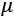from it. Then, the result is divided by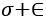, the sum of batch variance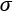and a small number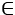, to prevent division by zero. Then, we use a linear transformation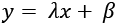to make sure that the normalizing effect is applied during training.

In this way,and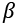are parameters that get optimized during the training phase in a similar way to any other layer. BatchNormalization has been proven as a very effective way to increase both the speed of training and accuracy, because it helps to prevent activations becoming either too small and vanishing or too big and exploding.

# Playing with Google Colab – CPUs, GPUs, and TPUs

Google offers a truly intuitive tool for training neural networks and for playing with TensorFlow (including 2.x) at no cost. You can find an actual Colab, which can be freely accessed, at https://colab.research.google.com/ and if you are familiar with Jupyter notebooks, you will find a very familiar web-based environment here. Colab stands for Colaboratory and it is a Google research project created to help disseminate machine learning education and research.

Let's see how it works, starting with the screenshot shown in Figure 32:

Figure 32: An example of notebooks in Colab

By accessing Colab, you can either check a listing of notebooks generated in the past or you can create a new notebook. Different versions of Python are supported.

When we create a new notebook, we can also select whether we want to run it on CPUs, GPUs, or in Google's TPUs as shown in Figure 25 (see Chapter 16, Tensor Processing Unit for more details on these):

Figure 33: Selecting the desired hardware accelerator (None, GPUs, TPUs) - first step

By accessing the Notebook settings option contained in the Edit menu (see Figure 33 and Figure 34), we can select the desired hardware accelerator (None, GPUs, TPUs). Google will allocate the resources at no cost, although they can be withdrawn at any time, for example during periods of particularly heavy load. In my experience, this is a very rare event and you can access colab pretty much any time. However, be polite and do not do something like start mining bitcoins at no cost – you will almost certainly get evicted!

Figure 34: Selecting the desired hardware accelerator (None, GPUs, TPUs) - second step

The next step is to insert your code (see Figure 35) in the appropriate colab notebook cells and voila! You are good to go. Execute the code and happy deep learning without the hassle of buying very expensive hardware to start your experiments! Figure 35 contains an example of code in a Google notebook:

Figure 35: An example of code in a notebook

# Sentiment analysis

What is the code we used to test colab? It is an example of sentiment analysis developed on top of the IMDb dataset. The IMDb dataset contains the text of 50,000 movie reviews from the Internet Movie Database. Each review is either positive or negative (for example, thumbs up or thumbs down). The dataset is split into 25,000 reviews for training and 25,000 reviews for testing. Our goal is to build a classifier that is able to predict the binary judgment given the text. We can easily load IMDb via `tf.keras` and the sequences of words in the reviews have been converted to sequences of integers, where each integer represents a specific word in a dictionary. We also have a convenient way of padding sentences to `max_len`, so that we can use all sentences, whether short or long, as inputs to a neural network with an input vector of fixed size (we will look at this requirement in more detail in Chapter 8, Recurrent Neural Networks):

``````import tensorflow as tf
from tensorflow.keras import datasets, layers, models, preprocessing
import tensorflow_datasets as tfds
max_len = 200
n_words = 10000
dim_embedding = 256
EPOCHS = 20
BATCH_SIZE = 500
(X_train, y_train), (X_test, y_test) = datasets.imdb.load_data(num_words=n_words)
# Pad sequences with max_len.
X_train = preprocessing.sequence.pad_sequences(X_train, maxlen=max_len)
X_test = preprocessing.sequence.pad_sequences(X_test, maxlen=max_len)
return (X_train, y_train), (X_test, y_test)
``````

Now let's build a model. We are going to use a few layers that will be explained in detail in Chapter 8, Recurrent Neural Networks. For now, let's assume that the `Embedding()` layer will map the sparse space of words contained in the reviews into a denser space. This will make computation easier. In addition, we will use a `GlobalMaxPooling1D()` layer, which takes the maximum value of either feature vector from each of the `n_words` features. In addition, we have two `Dense()` layers. The last one is made up of one single neuron with a sigmoid activation function for making the final binary estimation:

``````def build_model():
model = models.Sequential()
# Input: - eEmbedding Layer.
# The model will take as input an integer matrix of size (batch,     # input_length).
# The model will output dimension (input_length, dim_embedding).
# The largest integer in the input should be no larger
# than n_words (vocabulary size).
dim_embedding, input_length=max_len))
# Takes the maximum value of either feature vector from each of     # the n_words features.
return model
``````

Now we need to train our model, and this piece of code is very similar to what we did with MNIST. Let's see:

``````(X_train, y_train), (X_test, y_test) = load_data()
model = build_model()
model.summary()
model.compile(optimizer = "adam", loss = "binary_crossentropy",
metrics = ["accuracy"]
)
score = model.fit(X_train, y_train,
epochs = EPOCHS,
batch_size = BATCH_SIZE,
validation_data = (X_test, y_test)
)
score = model.evaluate(X_test, y_test, batch_size=BATCH_SIZE)
print("\nTest score:", score)
print('Test accuracy:', score)
``````

Let's see the network and then run a few iterations:

Figure 36: The results of the network following a few iterations

As shown in the following image, we reach the accuracy of 85%, which is not bad at all for a simple network:

Figure 37: Testing the accuracy of a simple network

# Hyperparameter tuning and AutoML

The experiments defined above give some opportunities for fine-tuning a net. However, what works for this example will not necessarily work for other examples. For a given net, there are indeed multiple parameters that can be optimized (such as the number of hidden neurons, `BATCH_SIZE`, number of epochs, and many more depending on the complexity of the net itself). These parameters are called "hyperparameters" to distinguish them from the parameters of the network itself, that is, the values of the weights and biases.

Hyperparameter tuning is the process of finding the optimal combination of those hyperparameters that minimize cost functions. The key idea is that if we have n hyperparameters, then we can imagine that they define a space with n dimensions and the goal is to find the point in this space that corresponds to an optimal value for the cost function. One way to achieve this goal is to create a grid in this space and systematically check the value assumed by the cost function for each grid vertex. In other words, the hyperparameters are divided into buckets and different combinations of values are checked via a brute force approach.

If you think that this process of fine-tuning the hyperparameters is manual and expensive, then you are absolutely right! However, during the last few years we have seen significant results in AutoML, a set of research techniques aiming at both automatically tuning hyperparameters and searching automatically for optimal network architecture. We will discuss more about this in Chapter 14, An introduction to AutoML.

# Predicting output

Once a net is trained, it can of course be used for making predictions. In TensorFlow this is very simple. We can use the method:

``````# Making predictions.
predictions = model.predict(X)
``````

For a given input, several types of output can be computed, including a method `model.evaluate()` used to compute the loss values, a method `model.predict_classes()` used to compute category outputs, and a method `model.predict_proba()` used to compute class probabilities.

# A practical overview of backpropagation

Multi-layer perceptrons learn from training data through a process called backpropagation. In this section, we will cover the basics while more details can be found in Chapter 15, The Math behind Deep Learning. The process can be described as a way of progressively correcting mistakes as soon as they are detected. Let's see how this works.

Remember that each neural network layer has an associated set of weights that determine the output values for a given set of inputs. Additionally, remember that a neural network can have multiple hidden layers.

At the beginning, all the weights have some random assignment. Then, the net is activated for each input in the training set: values are propagated forward from the input stage through the hidden stages to the output stage where a prediction is made. Note that we've kept Figure 38 simple by only representing a few values with green dotted lines but in reality all the values are propagated forward through the network:

Figure 38: Forward step in backpropagation

Since we know the true observed value in the training set, it is possible to calculate the error made in prediction. The key intuition for backtracking is to propagate the error back (see Figure 39), using an appropriate optimizer algorithm such as gradient descent to adjust the neural network weights with the goal of reducing the error (again, for the sake of simplicity, only a few error values are represented here):

Figure 39: Backward step in backpropagation

The process of forward propagation from input to output and the backward propagation of errors is repeated several times until the error gets below a predefined threshold. The whole process is represented in Figure 40:

Figure 40: Forward propagation and backward propagation

The features represent the input, and the labels are used here to drive the learning process. The model is updated in such a way that the loss function is progressively minimized. In a neural network, what really matters is not the output of a single neuron but the collective weights adjusted in each layer. Therefore, the network progressively adjusts its internal weights in such a way that the prediction increases the number of correctly forecasted labels. Of course, using the right set of features and having quality labeled data is fundamental in order to minimize the bias during the learning process.

# What have we learned so far?

In this chapter we have learned the basics of neural networks. More specifically, what a perceptron and what a multi-layer perceptron is, how to define neural networks in TensorFlow 2.0, how to progressively improve metrics once a good baseline is established, and how to fine-tune the hyperparameter space. In addition to that, we also have an intuitive idea of what some useful activation functions (sigmoid and ReLU) are, and how to train a network with backprop algorithms based on either gradient descent, SGD, or more sophisticated approaches such as Adam and RMSProp.

# Towards a deep learning approach

While playing with handwritten digit recognition, we came to the conclusion that the closer we get to the accuracy of 99%, the more difficult it is to improve. If we want more improvement, we definitely need a new idea. What are we missing? Think about it.

The fundamental intuition is that in our examples so far, we are not making use of the local spatial structure of images. In particular, this piece of code transforms the bitmap representing each written digit into a flat vector where the local spatial structure (the fact that some pixels are closer to each other) is gone:

``````# X_train is 60000 rows of 28x28 values; we  --> reshape it as in # 60000 x 784.
X_train = X_train.reshape(60000, 784)
X_test = X_test.reshape(10000, 784)
``````

However, this is not how our brain works. Remember that our vision is based on multiple cortex levels, each one recognizing more and more structured information, still preserving the locality. First, we see single pixels, then from those, we recognize simple geometric forms, and then more and more sophisticated elements such as objects, faces, human bodies, animals, and so on.

In Chapter 4, Convolutional Neural Networks we will see that a particular type of deep learning network, known as a Convolutional Neural Network (in short, CNN) has been developed by taking into account both the idea of preserving the local spatial structure in images (and more generally, in any type of information that has a spatial structure) and the idea of learning via progressive levels of abstraction: with one layer you can only learn simple patterns, with more than one layer you can learn multiple patterns. Before discussing CNNs, we need to discuss some aspects of TensorFlow architecture and have a practical introduction to a few additional machine learning concepts. This will be the topic of the upcoming chapters.

# References

1. F. Rosenblatt, The perceptron: a probabilistic model for information storage and organization in the brain, Psychol. Rev., vol. 65, pp. 386–408, Nov. 1958.
2. P. J. Werbos, Backpropagation through time: what it does and how to do it, Proc. IEEE, vol. 78, pp. 1550–1560, 1990.
3. G. E. Hinton, S. Osindero, and Y.-W. Teh, A fast learning algorithm for deep belief nets, Neural Comput., vol. 18, pp. 1527–1554, 2006.
4. J. Schmidhuber, Deep Learning in Neural Networks: An Overview, Neural networks : Off. J. Int. Neural Netw. Soc., vol. 61, pp. 85–117, Jan. 2015.
5. S. Leven, The roots of backpropagation: From ordered derivatives to neural networks and political forecasting, Neural Networks, vol. 9, Apr. 1996.
6. D. E. Rumelhart, G. E. Hinton, and R. J. Williams, Learning representations by back-propagating errors, Nature, vol. 323, Oct. 1986.
7. S. Herculano-Houzel, The Human Brain in Numbers: A Linearly Scaled-up Primate Brain, Front. Hum. Neurosci, vol. 3, Nov. 2009.
8. Hornick, Multilayer feedforward networks are universal approximators, Neural Networks Volume 2, Issue 5, 1989, Pages 359-366.
9. Vapnik, The Nature of Statistical Learning Theory, Book, 2013.
10. Sutskever, I., Martens, J., Dahl, G., Hinton, G., On the importance of initialization and momentum in deep learning, 30th International Conference on Machine Learning, ICML 2013.

### Latest Reviews

(6 reviews total)